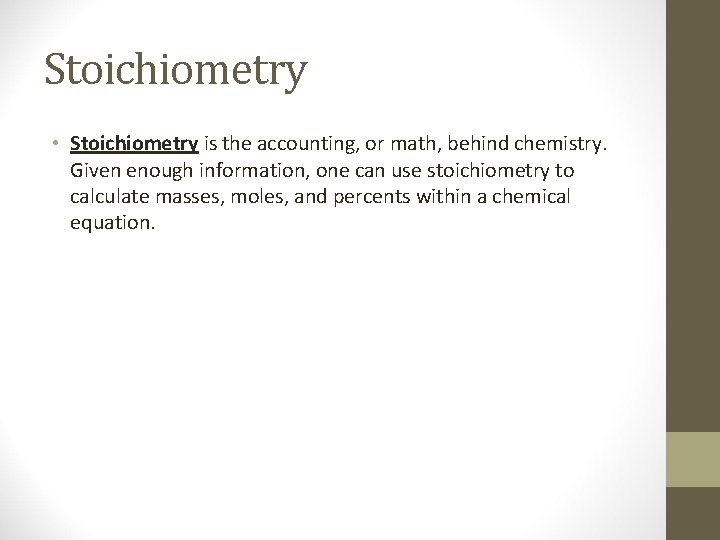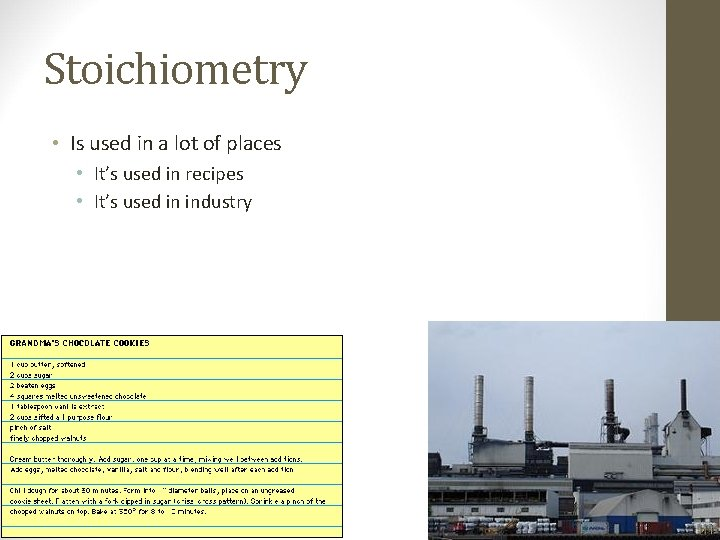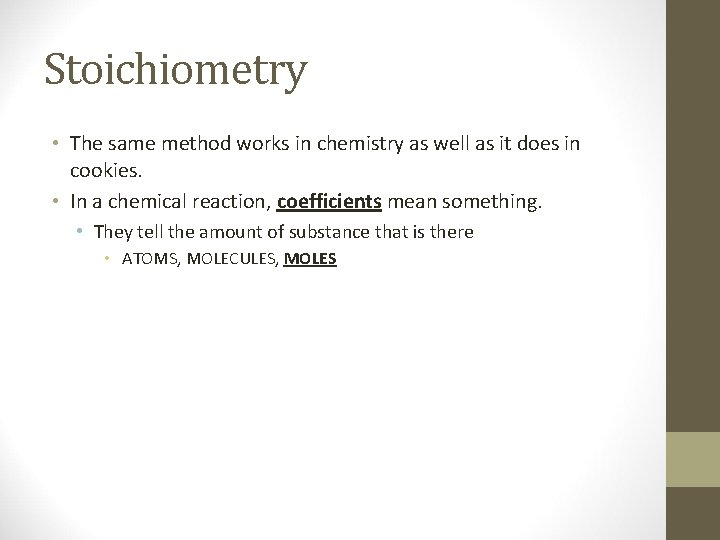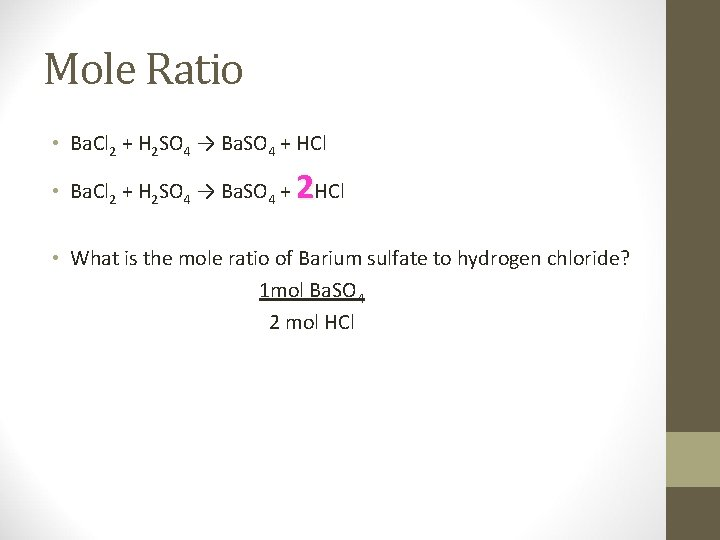# Stoichiometry Ch 9 Stoichiometry Stoichiometry is the accounting

• Slides: 7Stoichiometry Ch 9Stoichiometry • Stoichiometry is the accounting, or math, behind chemistry. Given enough information, one can use stoichiometry to calculate masses, moles, and percents within a chemical equation.Stoichiometry • Is used in a lot of places • It’s used in recipes • It’s used in industryStoichiometry • The same method works in chemistry as well as it does in cookies. • In a chemical reaction, coefficients mean something. • They tell the amount of substance that is there • ATOMS, MOLECULES, MOLESMole Ratio • Ba. Cl 2 + H 2 SO 4 → Ba. SO 4 + HCl 2 • Ba. Cl 2 + H 2 SO 4 → Ba. SO 4 + HCl • What is the mole ratio of Barium sulfate to hydrogen chloride? 1 mol Ba. SO 4 2 mol HClDoing stoichiometry: the steps • 1. Convert mass of given to moles. • 2. Use mole ratio to convert moles of given to moles of unknown. • 3. Convert moles of unknown to mass of unknown. STEP 1 STEP 2 mass of GIVEN 1 mol GIVEN Molar Mass of GIVEN STEP 3 moles of UNKNOWN from Molar Mass of UNKNOWN Balanced Eqn moles of GIVEN from Balanced Eqn 1 mol UNKNOWN